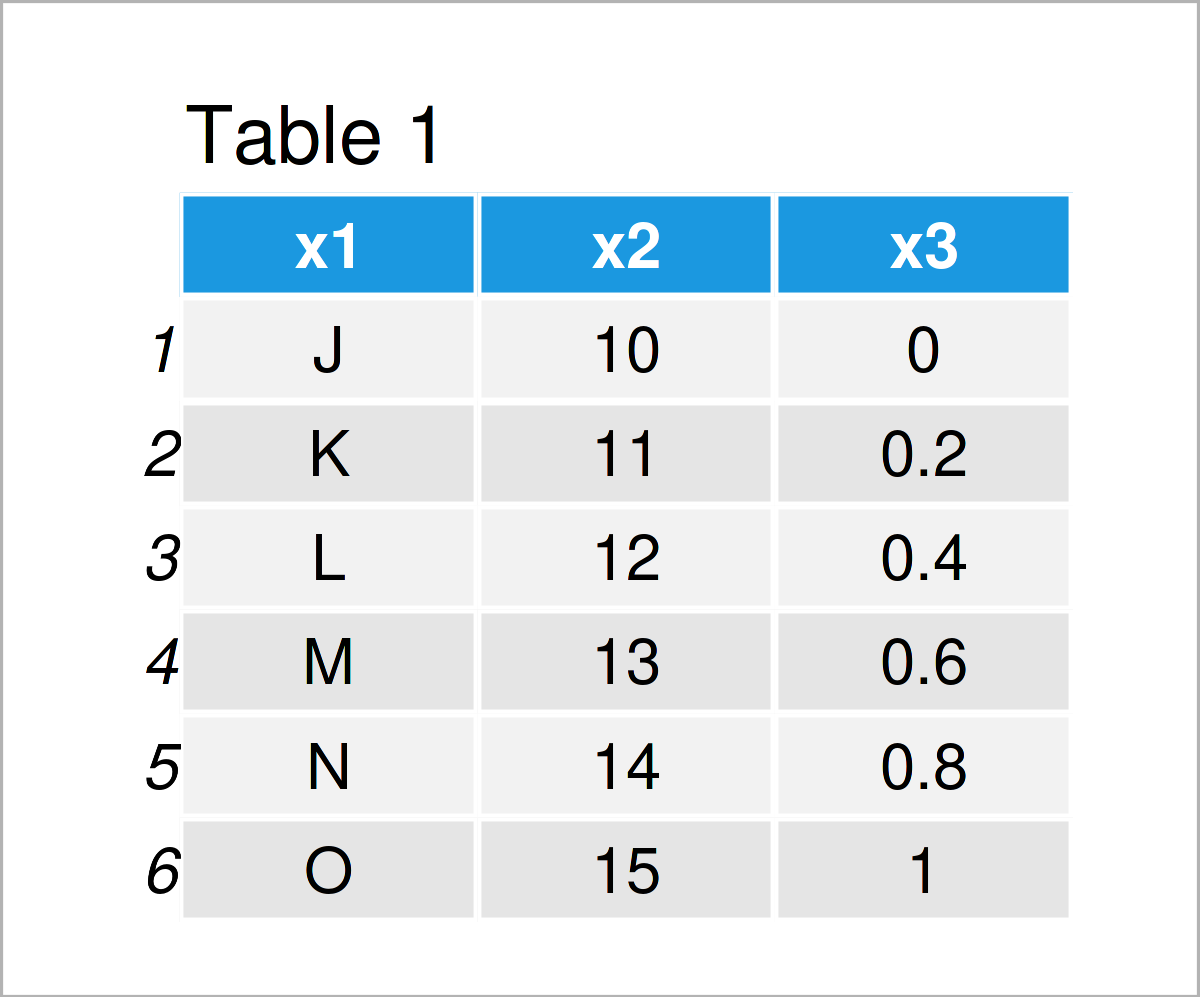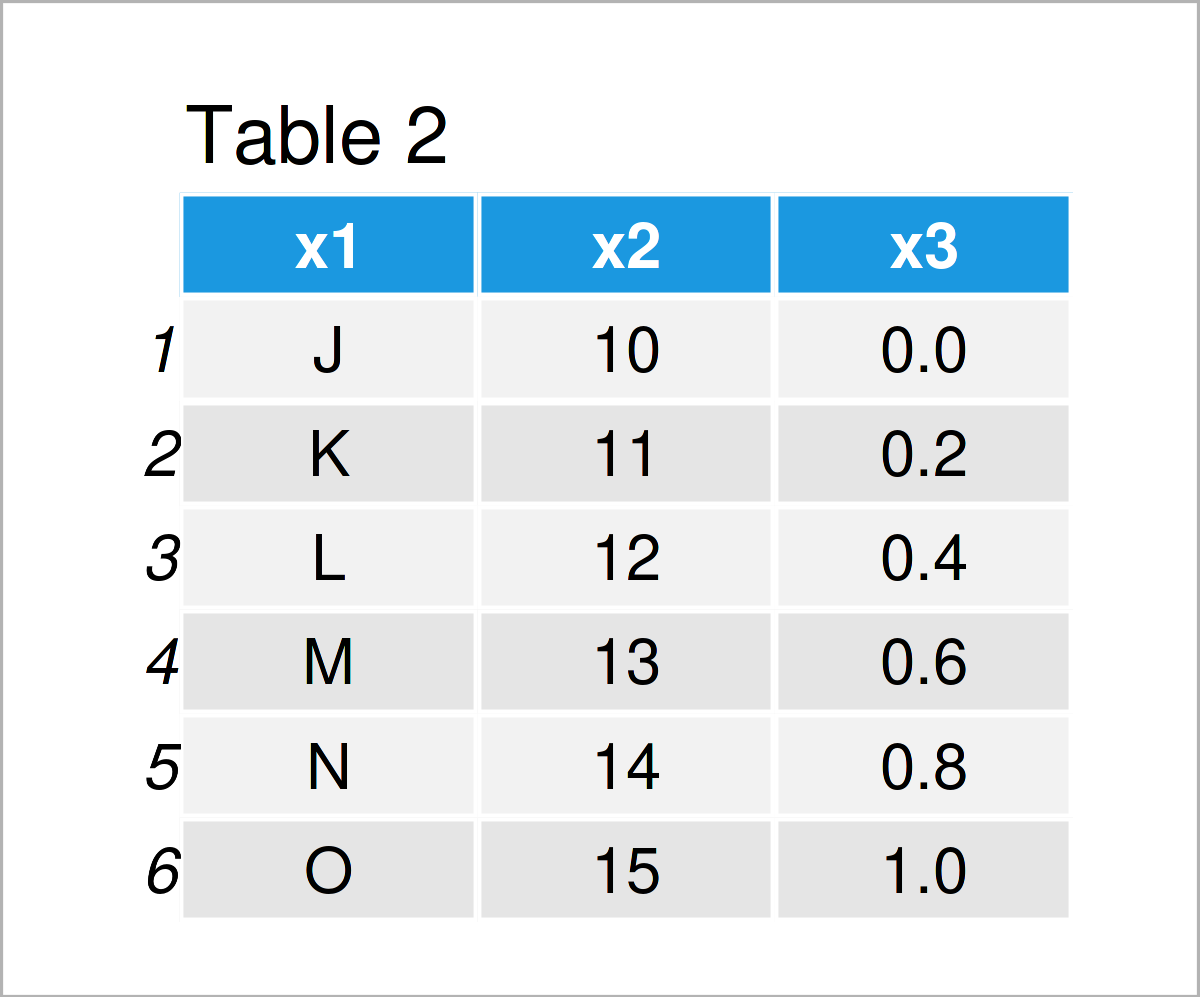# Change Classes of Data Frame Columns Automatically in R (Example)

In this R tutorial you’ll learn how to convert all data frame columns to the appropriate data type.

Let’s just jump right in:

## Creation of Example Data

The following data will be used as a basis for this R programming tutorial.

```data <- data.frame(x1 = LETTERS[10:15], # Create example data x2 = as.character(10:15), x3 = as.character(seq(0, 1, 0.2))) data # Print example data```As you can see based on Table 1, our example data is a data frame constructed of six rows and three columns.

Let’s test the classes of each variable in our data frame:

```sapply(data, class) # Return classes of columns # x1 x2 x3 # "character" "character" "character"```

As you can see, all columns in our data frame have the character class, even though the columns x2 and x3 contain integers and numerics.

Let’s change that!

## Example: Automatically Modify Column Classes Using type.convert() Function

This example explains how to find the best class for each data frame variable automatically.

For this task, we can use the type.convert function as shown below:

```data_new <- type.convert(data, as.is = TRUE) # Modify column classes data_new # Print updated data frame```After executing the previous R programming code the new data frame shown in Table 2 has been created. At this point, we cannot see any major differences compared to the input data frame.

However, once we check the classes of the variables in our data frame once again, the changes get obvious:

```sapply(data_new, class) # Check column classes of updated data # x1 x2 x3 # "character" "integer" "numeric"```

As you can see, the data classes of the columns x2 and x3 have been changed to integer and numeric. Looks good!

Please note that the code in this example is based on this Stack Overflow thread. Thanks to the commenters in this thread!

## Video, Further Resources & Summary

I have recently released a video on my YouTube channel, which illustrates the R programming syntax of this page. You can find the video instruction below:

Furthermore, you might want to have a look at some of the other R tutorials on my homepage.

At this point you should know how to change the classes of all data frame columns to the appropriate class in R programming. Let me know in the comments, in case you have additional questions. In addition, don’t forget to subscribe to my email newsletter to receive updates on the newest articles.

Subscribe to the Statistics Globe Newsletter

•Guia
June 15, 2022 9:52 pm

When I imported my CSV, some columns which are supposed to “integer” or “double” were classed as ‘characters’. I was manually updating the classes of each column of my data frame by creating a list of all the classes. and then using apply, cols, or col_types. Good thing I found your article! This definitely made everything easier.